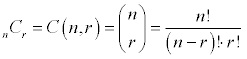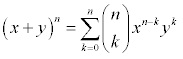#### Theory of Binomial Theorem

The Binomial Theorem:

Binomials rose to a power:

The binomial is a polynomial containing two terms. We are going to look at Binomial Expansion Theorem, a shortcut technique of raising a binomial to a power.

(x+y)0 = 1
(x+y)1 = x + y
(x+y)2 = x2 + 2xy + y2
(x+y)3 = x3 + 3x2y + 3xy2 + y3
(x+y)4 = x4 + 4x3y + 6x2y2 + 4xy3 + y4
(x+y)5 = x5 + 5x4y + 10x3y2 +10x2y3 + 5xy4 + y5

There are some things which you hopefully have observed after looking at the expansion:

a) There are (n+1) terms in the expansion of (x+y)n
b) The degree of each and every term is n.
c) The powers on x start with n and reduce to 0.
d) The powers on y start with 0 and rise to n.
e) Coefficients are symmetric.

Pascal's Triangle:

Pascal's Triangle introduced and named after the French mathematician Blaise Pascal is an easy way to determine the coefficients of expansion.

Each and every row in the triangle starts and ends with 1. Each and every element in the triangle is the sum of two elements instantly above it.
1
1   1
1   2   1
1   3   3   1
1   4   6   4   1
1   5  10  10   5   1
1   6  15  20  15   6   1
1   7  21  35  35  21   7   1

Combinations:

The brief summary to get you going with the Binomial Expansion Theorem is explained below.The combination is an arrangement of objects, without repetition, and order not being significant. The other definition of combination is the number of such arrangements which are possible.

The n and r in the formula signifies for the total number of objects to select from and the number of objects in the arrangement, correspondingly.

The fastest way, if unimaginative, is to employ the combination function on your calculator. On TI-82 and TI-83, it is found beneath the Math menu, Probability submenu and choice 3. Enter the value for n first, then in nCr notation, then the value for r.

Each and every element in Pascal's Triangle is a combination of n things. The value for r starts with zero and works its way up to the n. Or, since of symmetry, we could say it starts with n and works its way down to the 0.

Let's consider n = 4 row of the triangle.

4C0 = 1, 4C1 = 4, 4C2 = 6, 4C3 = 4, 4C4 = 1

Note that the 3rd term is the term with r = 2. That is, we start counting with 0. This will come to play later.

Binomial Expansion Theorem:

The Binomial Expansion Theorem can be written in the summation notation, where it is much compact and manageable.Keep in mind that as the lower limit of the summation starts with 0, the 7th term of the sequence is really the term if  k = 6.

Whenever you go to utilize the binomial expansion theorem, it is actually simpler to place the guidelines from the top of this page into practice. The x begins off to the nth power and goes down by one each time, the y begins off to the 0th power (not there) and rises by one each time. Coefficients are the combinations.

Binomial Expansion Example:

Expand (3x - 2y)5

Start off by figuring out coefficients. Keep in mind that such are the combinations of 5 things, k at a time, where k is either the power on x or the power on y (that is, combinations are symmetric, therefore it doesn't matter).

C(5,0) = 1; C(5,1) = 5; C(5,2) = 10; C(5,3) = 10; C(5,4) = 5; C(5,5) = 1

Now throw in 3x and -2y terms.

1(3x)5(-2y)0 + 5(3x)4(-2y)1 + 10(3x)3(-2y)2 + 10(3x)2(-2y)3 + 5(3x)1(-2y)4 + 1(3x)0(-2y)5

Raise the individual factors to their appropriate powers.

1(243x5)(1) + 5(81x4)(-2y) + 10(27x3)(4y2) + 10(9x2)(-8y3) + 5(3x)(16y4) + 1(1)(-32y5)

Simplify each of the term to get the final answer.

243x5 - 810x4y + 1080x3y2 - 720x2y3 + 240xy4 - 32y5

Finding the kth term:

Determine the 9th term in the expansion of (x-2y)13

As we start counting with 0, the 9th term is really going to be if k = 8. That is, the power on the x will 13 – 8 = 5 and the power on -2y will be 8. The coefficient is either C(13, 8) or C(13, 5), combinations are symmetric, therefore it doesn't matter.

C(13, 8) * (x)5 (-2y)8 = 1287 (x5) (256y8) = 329472x5y8

Latest technology based Algebra Online Tutoring Assistance

Tutors, at the www.tutorsglobe.com, take pledge to provide full satisfaction and assurance in Algebra help via online tutoring. Students are getting 100% satisfaction by online tutors across the globe. Here you can get homework help for Algebra, project ideas and tutorials. We provide email based Algebra help. You can join us to ask queries 24x7 with live, experienced and qualified online tutors specialized in Algebra. Through Online Tutoring, you would be able to complete your homework or assignments at your home. Tutors at the TutorsGlobe are committed to provide the best quality online tutoring assistance for Algebra Homework help and assignment help services. They use their experience, as they have solved thousands of the Algebra assignments, which may help you to solve your complex issues of Algebra. TutorsGlobe assure for the best quality compliance to your homework. Compromise with quality is not in our dictionary. If we feel that we are not able to provide the homework help as per the deadline or given instruction by the student, we refund the money of the student without any delay.